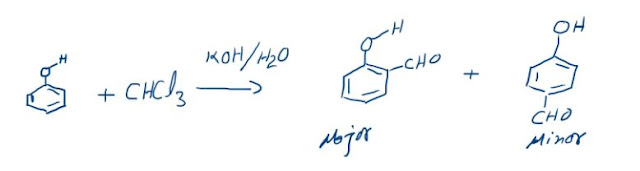## Hybridization

(a) The concept of hybridization was introduced by Pauling.
(b) Hybridization is defined as the intermixing of dissimilar orbitals of the same atom but having slightly different energies to form same number of new orbitals of equal energies and identical shapes.
(c) The new orbitals so formed are known as hybrid orbitals whose shape is similar to p orbital except that the two lobes are unequal in size i.e. one lobe is smaller while the other is bigger as shown below :

### Rules of Hybridization

(i) Only orbitals (atomic) of nearly same energy belonging to same atom or ion can take part in hybridization.
(ii) Number of the hybrid orbitals formed is always equal to number of atomic orbitals which have taken part in the process of hybridization.
(iii) Most of the hybrid orbitals are similar but they are not necessarily identical in shape. They may differ from one another in orientation in space.
(iv) Actually the orbitals which undergo hybridization and not the electrons.

### Types of hybridization

Depending upon the type and number of orbitals involved in intermixing, the hybridization can be of various types namely sp, sp2, sp3, sp3d, dsp2, sp3d2, sp3d3. The nature and number of orbitals involved in the above mentioned types of hybridization and their acquired shapes are dissociated as follows:
1. sp hybridization:
a) When one s and one p orbital belonging to the same main shell of an atom mix together to form two new equivalent orbitals, the type of hybridization is called sp hybridization or diagonal hybridization.
(b) The new orbitals formed are called sp hybrid orbitals.
(c) They are collinear with an angle of 180° as shown in figure.
(d) Each orbital has 50% s-character and 50% p-character.

2. sp2 hybridization:
a) When one s and two p-orbitals of the same shell of an atom mix to from three new equivalent orbitals, the type of hybridization is called sp2 hybridization or trigonal hybridization.
(b) The new orbitals formed are called sp2 hybrid orbitals.
(c) All the three hybrid orbitals remain in the same plane making an angle of 120° with one another as shown in figure.
(d) Each of the hybrid orbitals formed has 1/3rd s character and 2/3rd p-character.

3. sp3 hybridization:
a) When one s and three p-orbitals belonging to the same shell of an atom mix together to form four new equivalent orbitals the type of hybridization is called sp3 or tetrahedral hybridization.
(b) The new orbitals are called sp3 or tetrahedral orbitals.
(c) These are directed towards the four corners of a regular tetrahedron and make an angle of 109°28’ with one another as shown in figure.
(d) Each sp3 hybrid orbital has 25% s-character and 75% p-character.

4. sp3d hybridization:
This can be understood taking example of PCl5.
(a)         Electronic configuration of phosphorous in ground state

(c) Now there are five orbitals:
One s, three p (px, py, pz) and one ( dz2 -orbital) which are singly filled.
(d) These orbitals hybridize to yield a set of five sp3d hybrid orbitals.
(e) The resultant shape is trigonal bipyramidal.
(f) There are two sets of equivalent hybrid orbitals: one set consists of three coplanar (120°) equivalent equatorial e’ sp2 orbitals and the other consisting of two equivalent axial a dp orbitals as shown in figure

Shape of PCl5 molecule (Trigonal bipyramidal)

5. sp3d2 Hybridization:
This is explained by taking case of SF6
(a) In sulphur two electrons (one 3s and one 3p) are promoted to empty dz2 and dx2-y2 orbitals.
(b)Ground state configuration of S

These orbitals yield a set of six sp3d2 hybridized orbitals directed towards the corners of a regular octahedron as shown below.
Octahedral geometry of SF6 molecule

### The Reimer-Tiemann reaction: Reaction and its Mechanism

Reimer-Tiemann Reaction Phenols on reaction with chloroform in the presence of sodium hydroxide (or potassium hydroxide) solution give hydro...